### Computing the Weight of Evidence and Information Value

PROC HPBIN can compute the weight of evidence and the information value.

Weight of evidence (WOE) is a measure of how much the evidence supports or undermines a hypothesis. WOE measures the relative risk of an attribute of binning level. The value depends on whether the value of the target variable is a non-event or an event. An attribute’s WOE is defined as follows: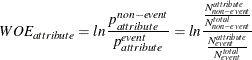The definitions of the quantities in the preceding formula are as follows:

•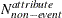: the number of non-event records that exhibit the attribute

•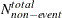: the total number of non-event records

•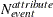: the number of event records that exhibit the attribute

•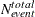: the total number of event records

To avoid an undefined WOE, an adjustment factor,, is used: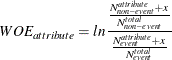You can use the WOEADJUST= option to specify a value between [0, 1] for. By default,is 0.5.

The information value (IV) is a weighted sum of the WOE of the characteristic’s attributes. The weight is the difference between the conditional probability of an attribute given an event and the conditional probability of that attribute given a non-event. In the following formula of IV,is the number of bins of a variable: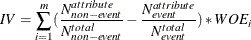An information value can be any real number. Generally speaking, the higher the information value, the more predictive a characteristic is likely to be.